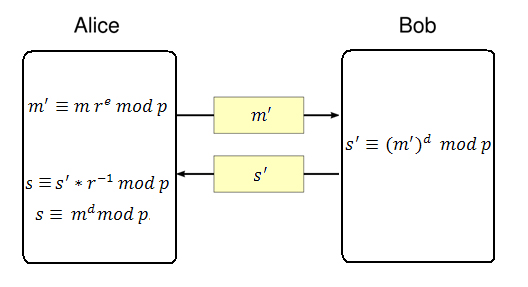## Posts

Showing posts from January, 2017

### Mandelbrot setThe Mandelbrot set and its complex beauty.

### RSA algorithmRSA is a practical public-key cryptographic algorithm, which is widely used on modern computers to communicate securely over large distances.

The acronym of the algorithm stands for Ron Rivest, Adi Shamir and Leonard Adleman, which first published the algorithm in 1978.

# Algorithm overviewChoose p and q as distinct prime numbersCompute n as n = p*qCompute \phi(n) as \phi(n) = (p-1) * (q-1)Choose e such that 1 < e < \phi(n) and e and \phi(n) are coprimeCompute the value of d as d ≡ e^(-1) mod \phi(n)Public key is (e, n)Private key is (d, n)The encryption of m as c, is c ≡ m^e mod nThe decryption of c as m, is m ≡ c^d mod n
# Generating p and q In order to generate a public and a private key, the algorithm requires two distinct prime numbers p and q, which are randomly chosen and should have, roughly, the same number of bits. By today standards, it is recommended that each prime number to have at least 2048 bits.

In Perl, there is a…

### InfinitesimalsIn this post we're going to take a look at what infinitesimals are and why they are important.
Infinitesimals are an abstract concept of very small values that are impossible to represent quantitatively in a finite system.

# Definition We define one infinitesimal as:

ε = lim_{n to \infty}\frac{1}{n}

with the inequality: ε > 0.

In general, the following inequalities hold true:

\frac{0}{n} < \frac{1}{n} < \frac{2}{n} < ... < \frac{n}{n}

as n -> \infty.

# Appearance The infinitesimals appear in some fundamental limits, one of which is the limit for the natural exponentiation function:

lim_{n to \infty}(1 + \frac{\x}{n})^n = \exp(\x)

Using our infinitesimal notation, we can rewrite the limit as:

lim_{n to \infty}(1 + ε*\x)^n = \exp(\x)

where, for x=1, we have:

lim_{n to \infty}(1 + ε)^n = \e.

# Debate There was (and, probably, still is) a debate in mathematics whether the following limit:

lim_{n to \infty}\frac{1}{n}

is 0 or greater than 0.

Con…